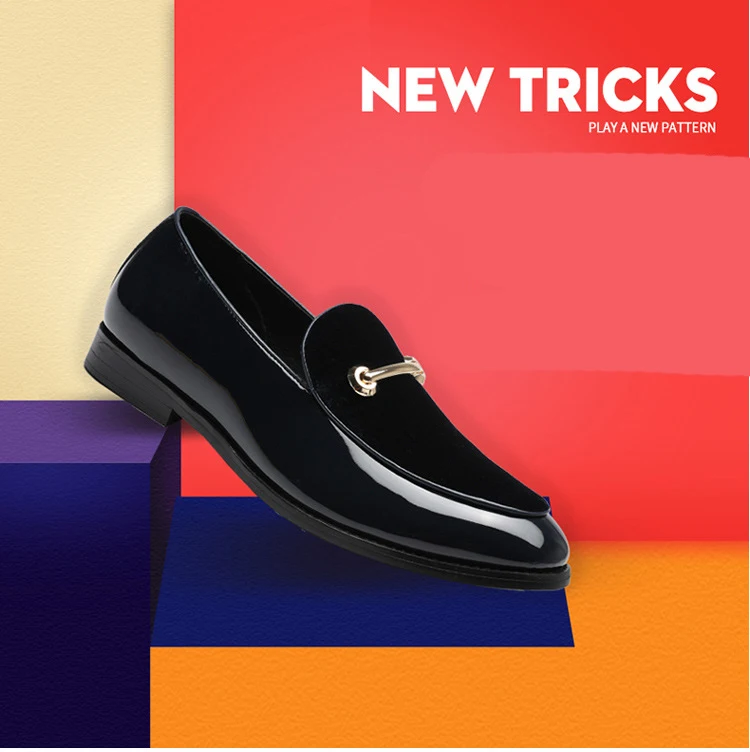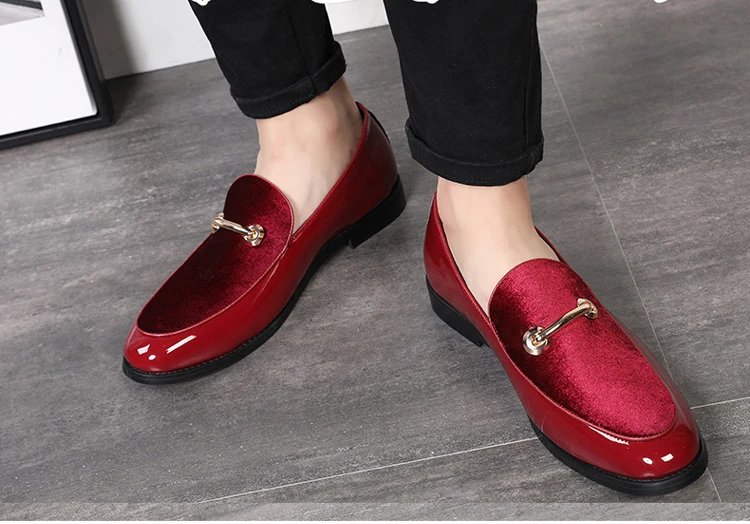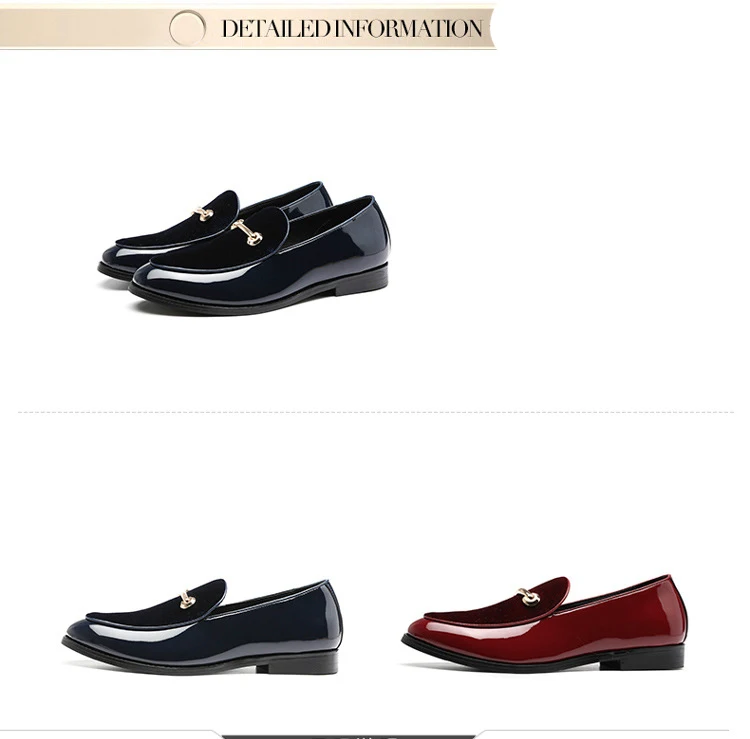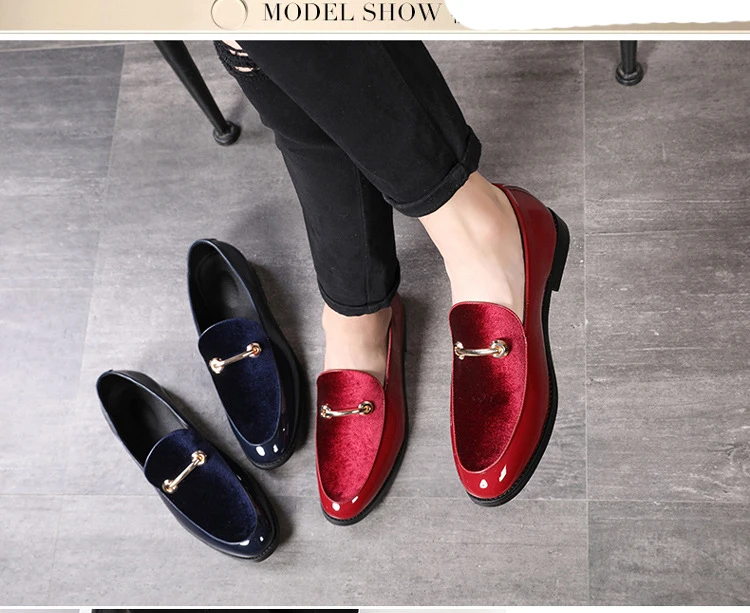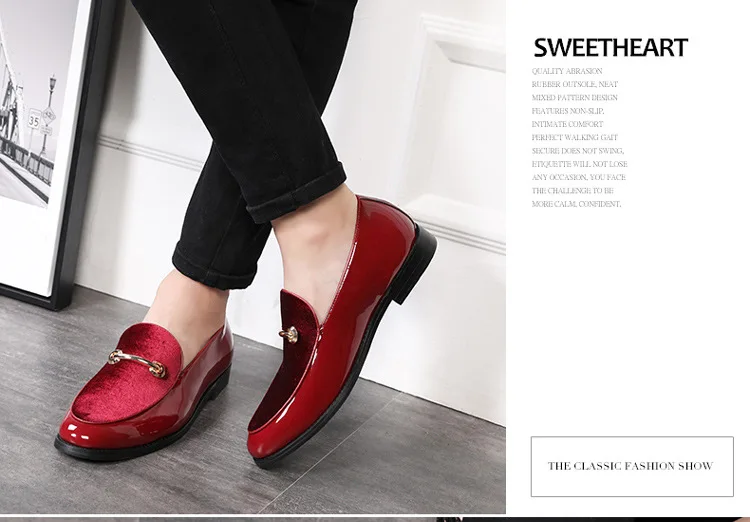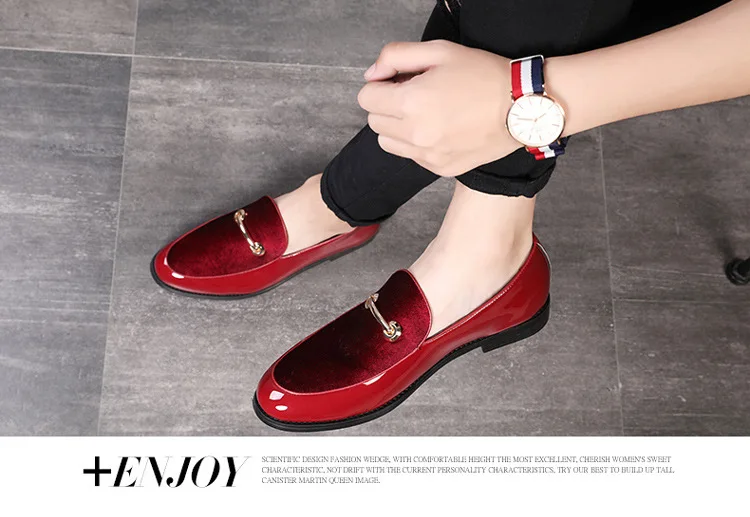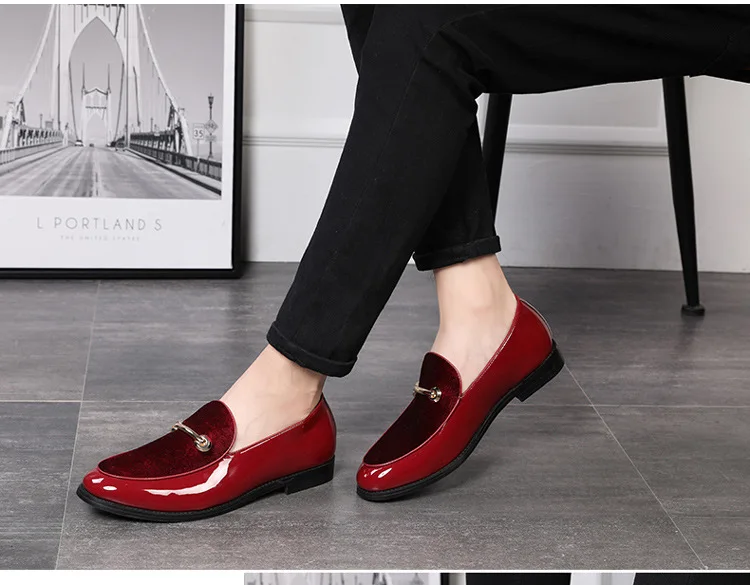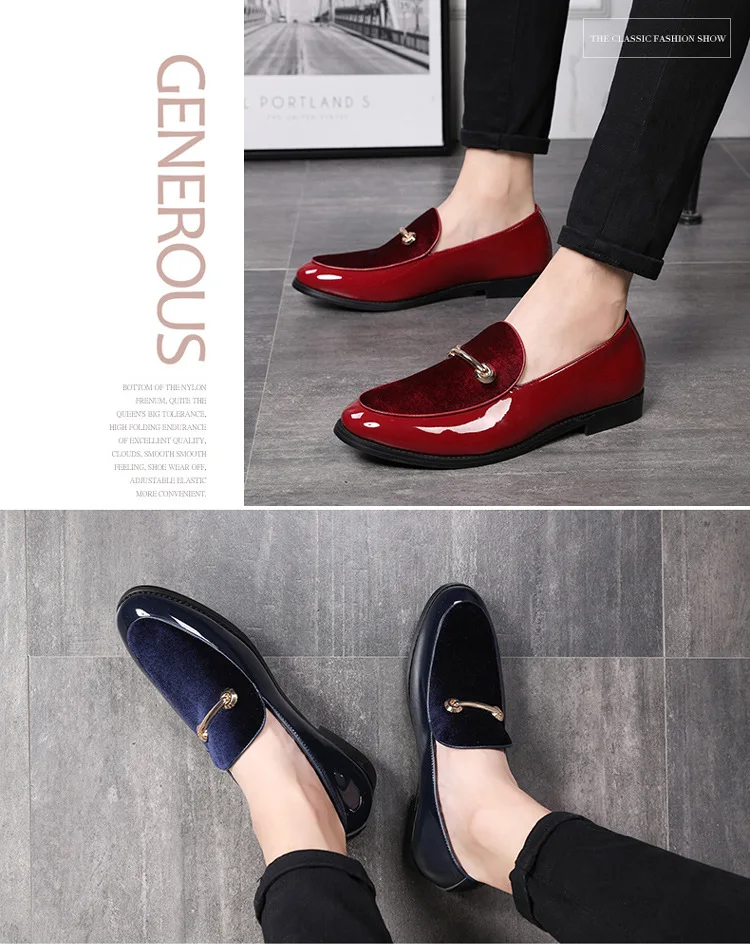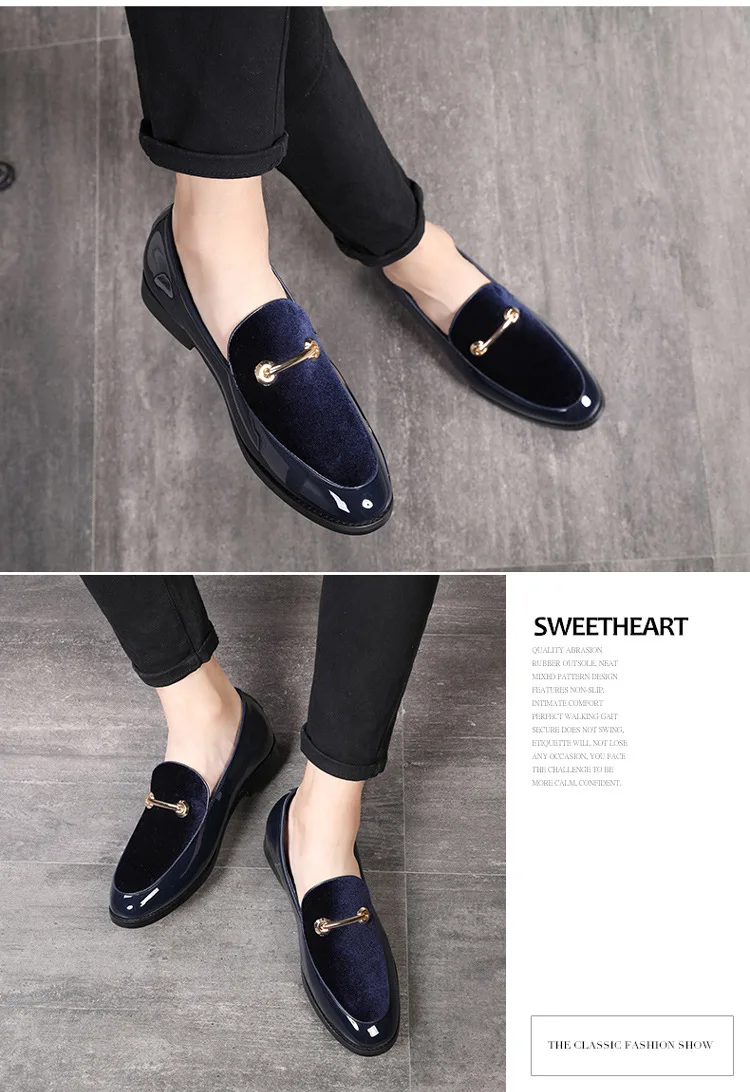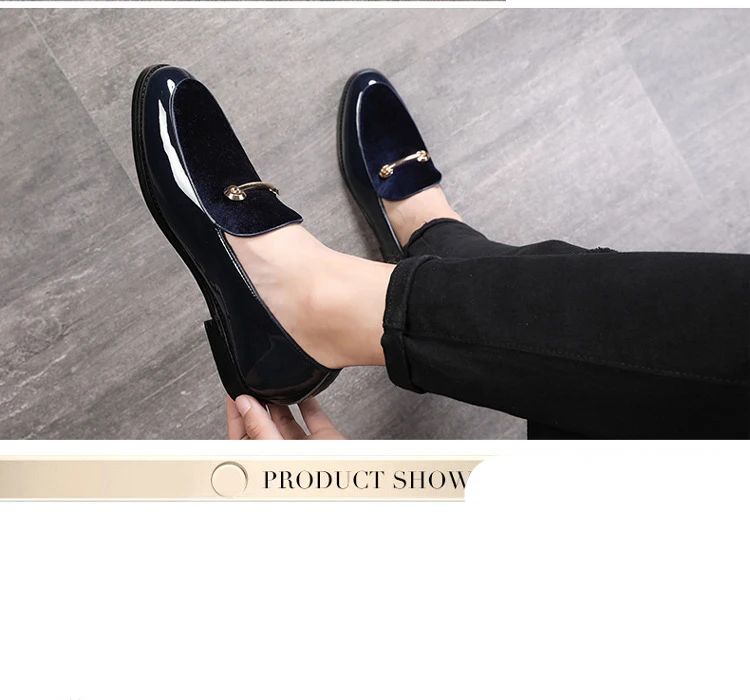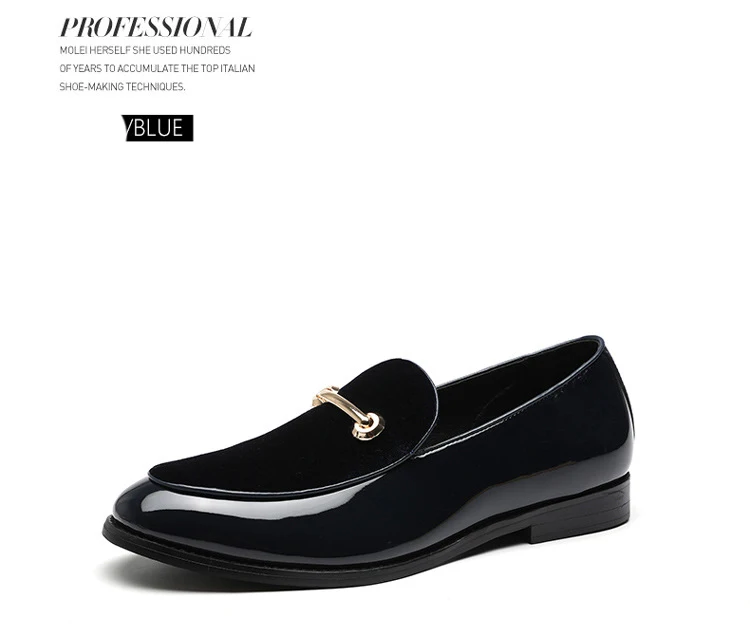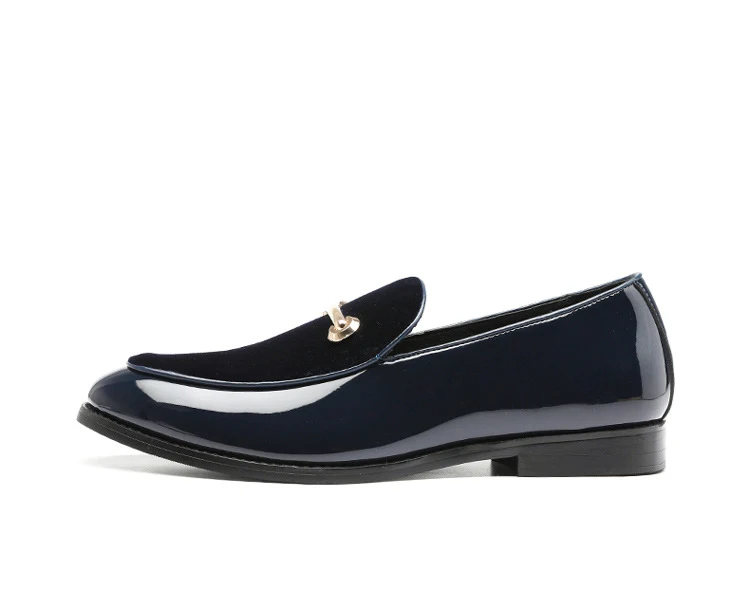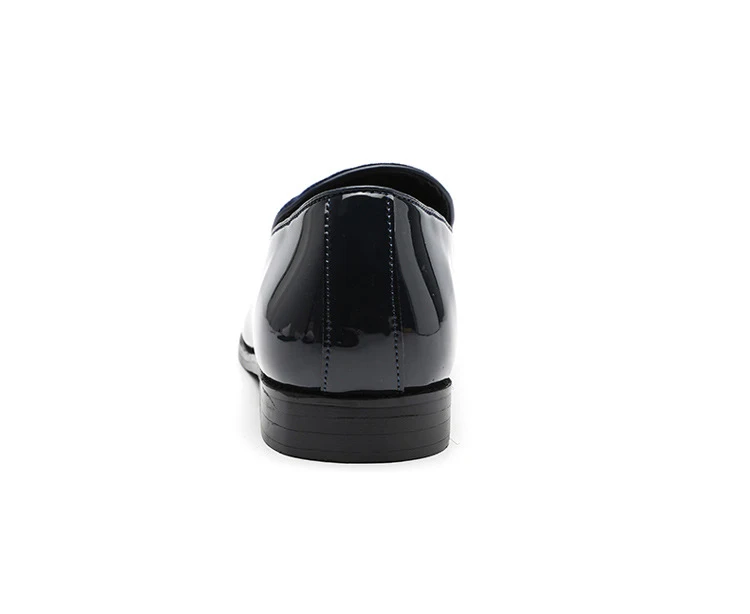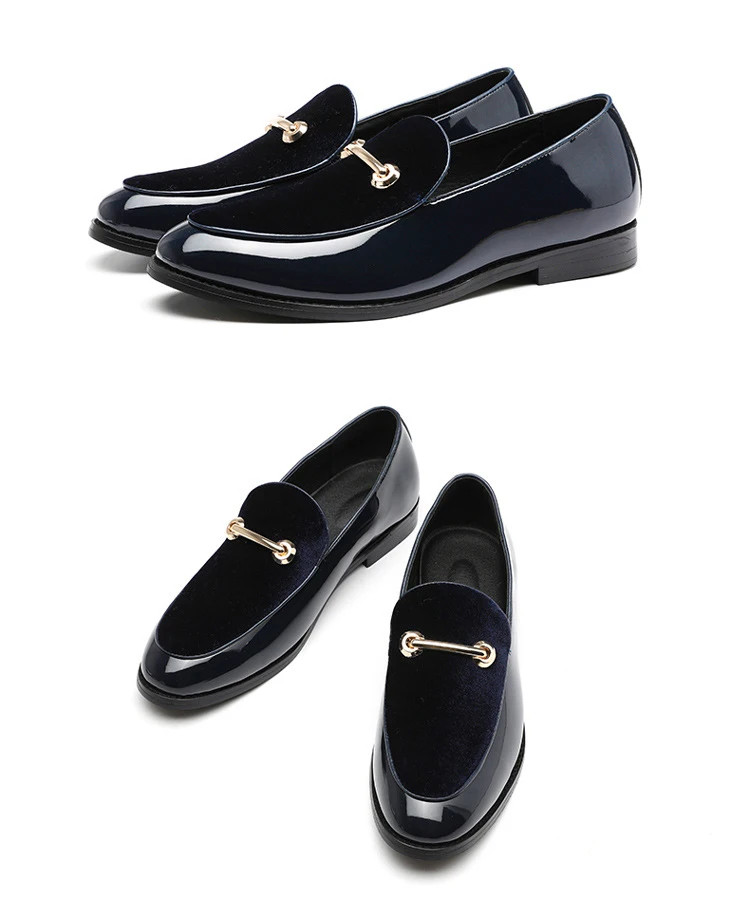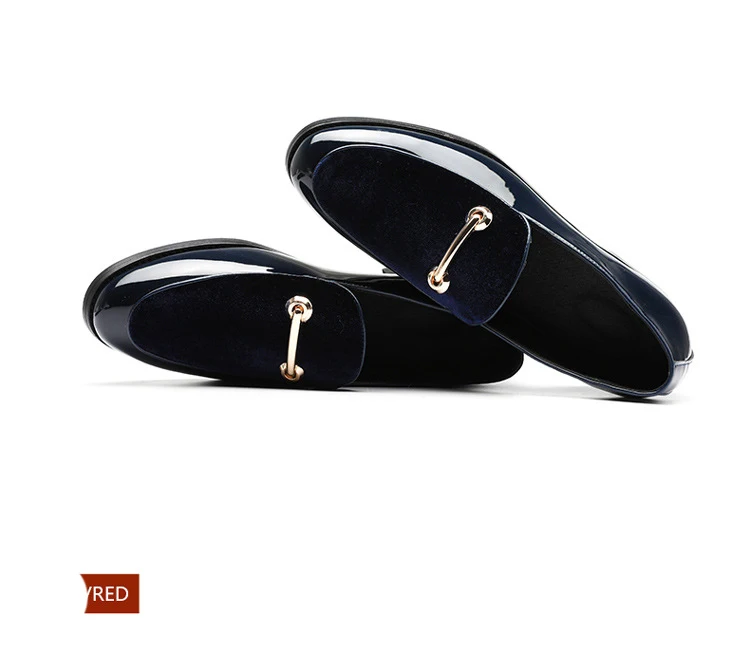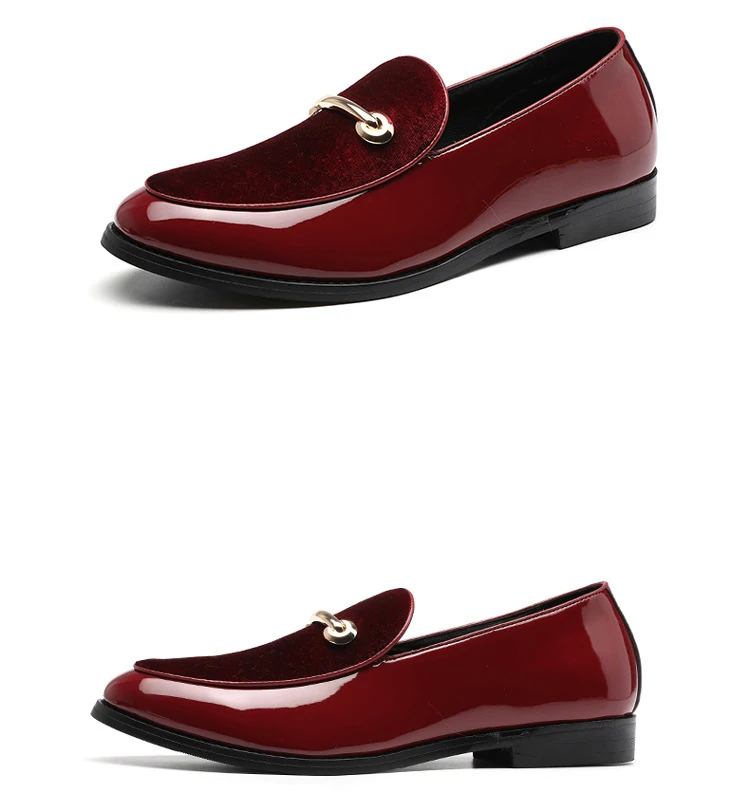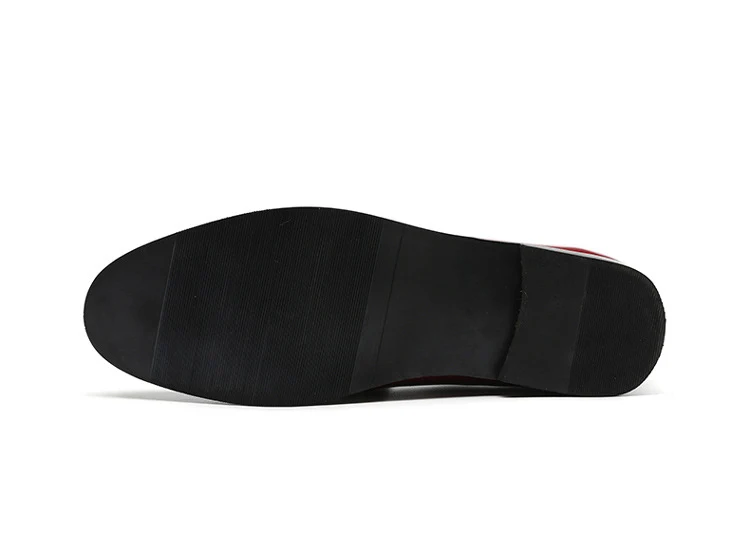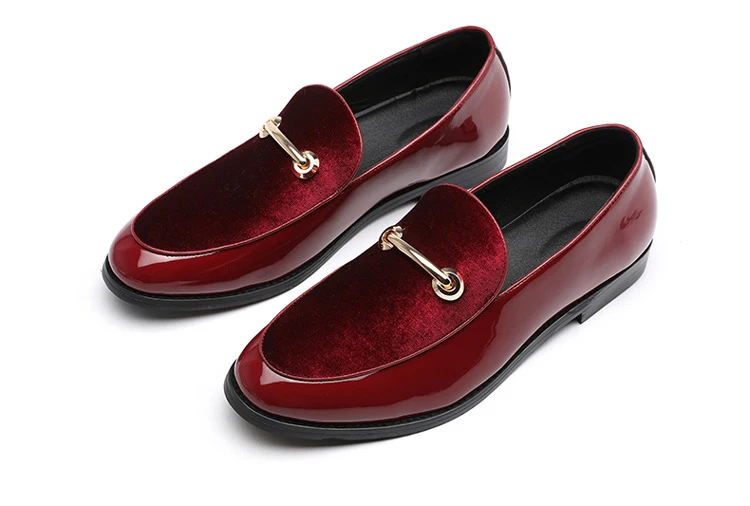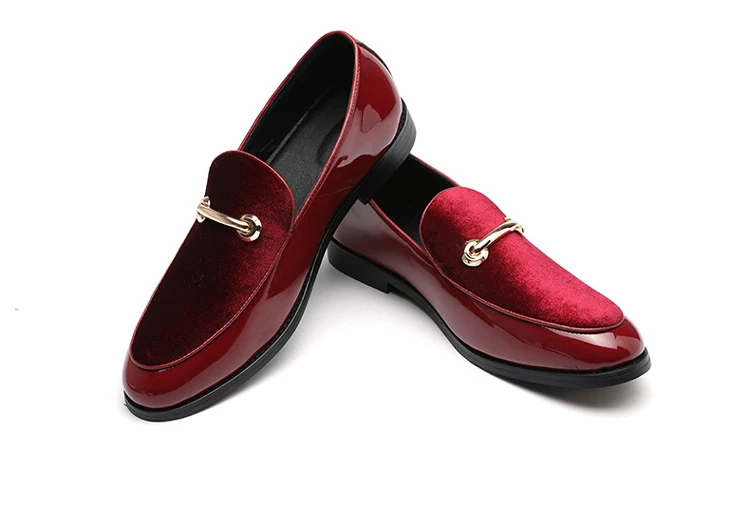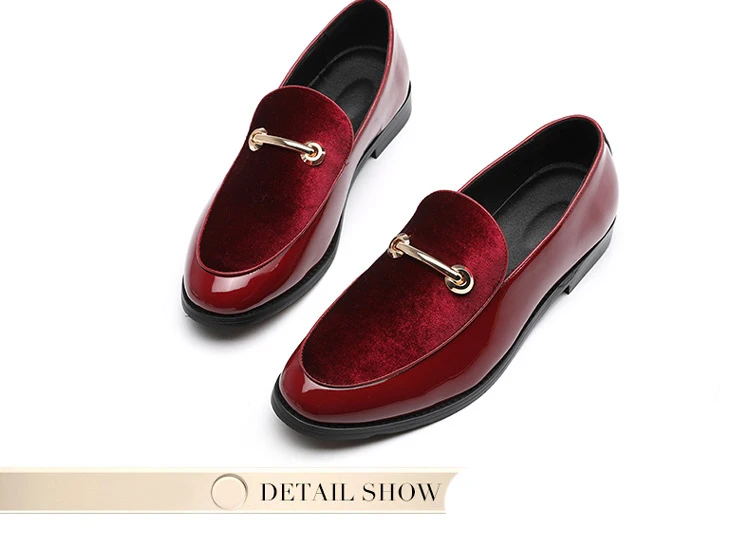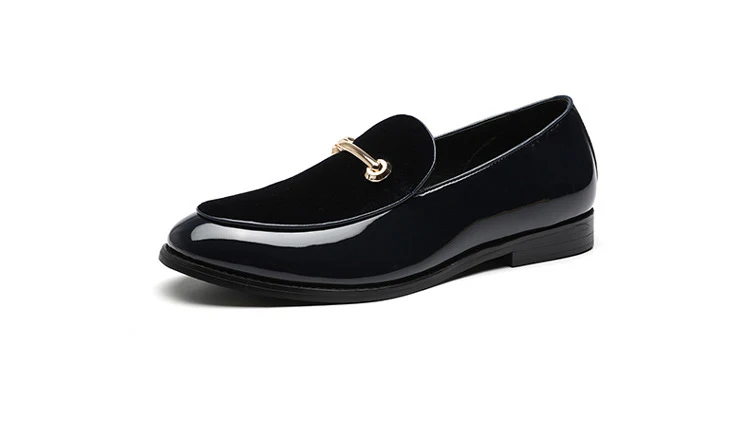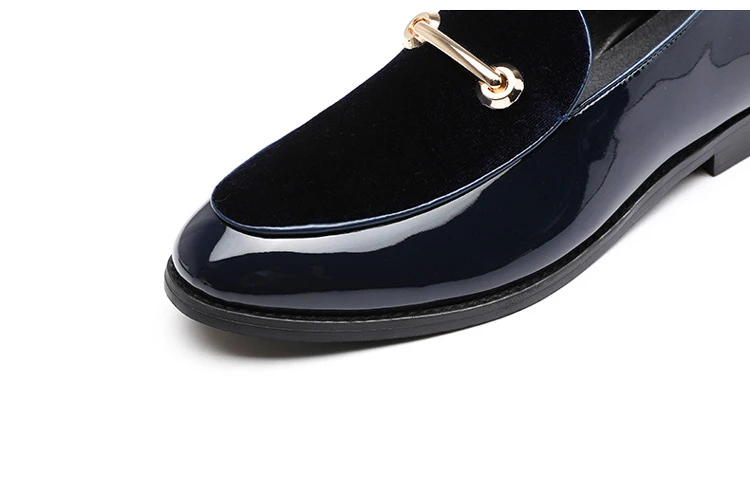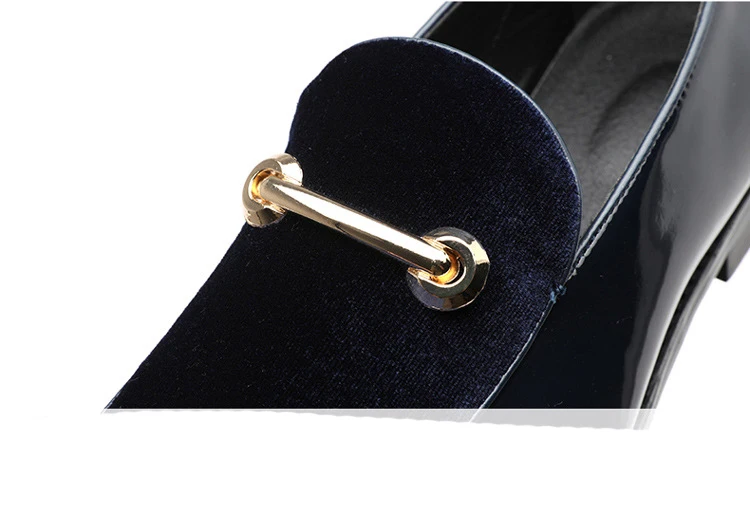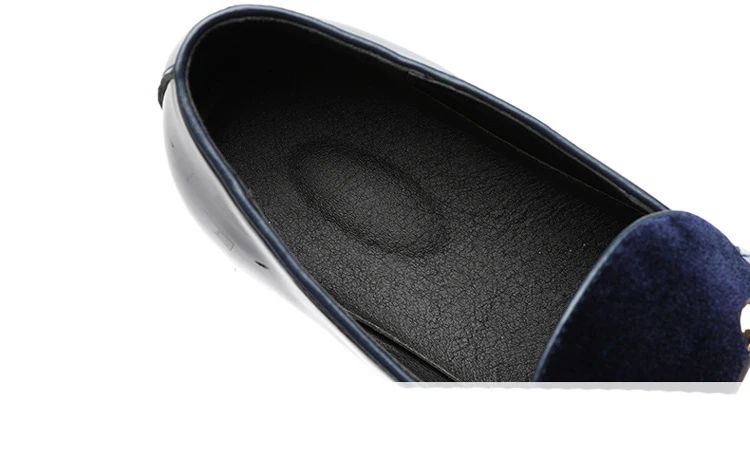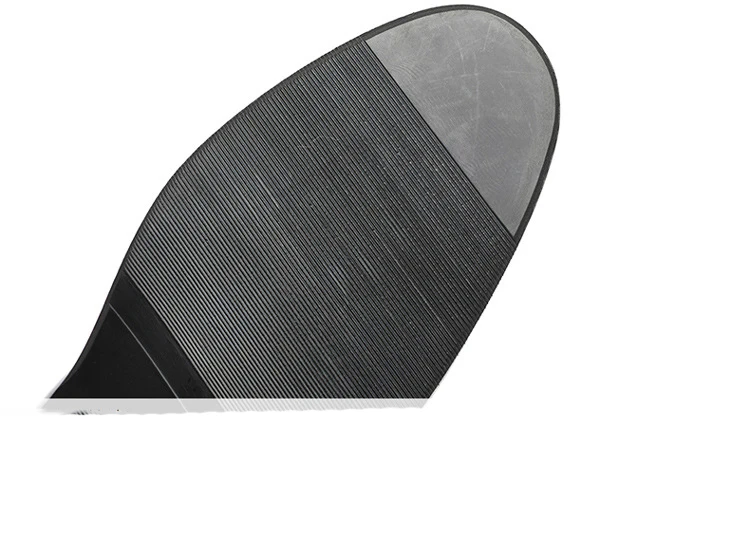# M-anxiu Fashion Pointed Toe Dress Shoes Men Loafers Patent Leather Oxford Shoes for Men Formal Mariage Wedding Shoes

34.93\$

 Color: Choose an optionBlackRed Shoe Size: Choose an option373839404142434445464748Clear
SKU: 11590 Categories: ,

Size:37,38,39,40,41,42,43,44,45,46,47,48

1,Note Our size is not standard US size, and it is only equal to foot length!

US5.5 =EU37=235mm=9.25in(bare feet length)

US6.0 =EU38=240mm=9.45in

US6.5 =EU39=245mm=9.65in

US7.0 =EU40=250mm=9.84in

US8.0 =EU41=255mm=10.04in

US8.5 =EU42=260mm=10.24in

US9.5 =EU43=265mm=10.43in

US10.0=EU44=270mm=10.63in

US11.0=EU45=275mm=10.83in

US12.0=EU46=280mm=11.02in

US12.5=EU47=285mm=11.22in

US13.0=EU48=290mm=11.42in